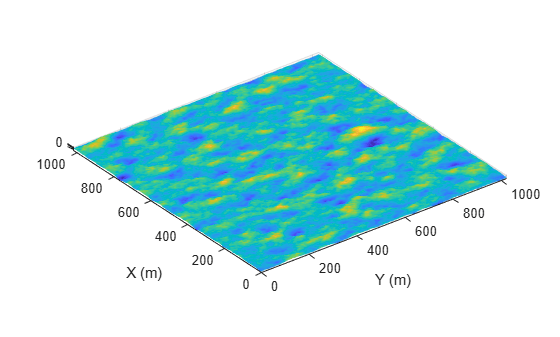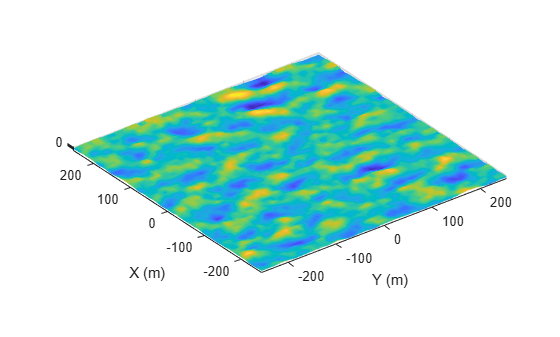# seaSpectrum

Sea surface omnidirectional motion spectrum model

Since R2022a

## Description

The `seaSpectrum` object creates a spectrum model for use in the `SpectralModel` property of the `SeaSurface` object.

## Creation

### Syntax

``seaspect = seaSpectrum``
``seaspect = seaSpectrum(Name = Value)``

### Description

````seaspect = seaSpectrum` creates a `seaSpectrum` object `seaspect` with default property values. The default sea surface spectrum and spreading function are based on the Elfouhaily model. The Elfouhaily model is an omnidirectional and wind-dependent spectrum. The wave spectrum consists of the frequency spectrum and angular spreading function. The spreading function is symmetric about the wind direction and has both wave number and wind speed dependence.```

example

````seaspect = seaSpectrum(Name = Value)` creates a `seaSpectrum` object with the specified property `Name` set to the specified `Value`. You can specify additional name-value pair arguments in any order as (```Name1 = Value1```, ..., `NameN = ValueN`).```

## Properties

expand all

Source of the omnidirectional wave spectrum, specified as `'Auto'` or `'Custom'`.

• Choosing `'Auto'` creates an Elfouhaily sea spectrum. The object offers properties that tailor the Elfouhaily sea spectrum.

• Choosing `'Custom'` allows you to specify the omnidirectional wave spectrum using the `CustomSpectrum` and `WaveVectorSpacing` properties.

Data Types: `char` | `string`

Sea surface resolution, specified as a positive scalar or as a 1-by-2 vector of positive values. The resolution vector takes the form ```[resolutionX resolutionY]``` where `resolutionX` and `resolutionY` denote the resolution in the x- and y- directions, respectively. If the resolution is a scalar, the x-resolution and y-resolution is the same.

The sea surface physical length is calculated as the difference of the limits of the `Boundary` property of the `SeaSurface` object in the x and y dimensions. The sea surface length has samples spaced by the `Resolution` property. The length should not be set below 0.02 m as the wave motion below 0.02 m is minimal. Units are in meters.

Data Types: `double`

Omnidirectional wave spectrum, specified as an M-by-N matrix. M and N dictate the inverse fast Fourier transfer (IFFT) length when returning the elevation data of the sea surface in the x and y dimensions, respectively.

The resolution for the custom case is calculated as Resolution = surface length./size(`CustomSpectrum`), where surface length is the sea surface physical length, calculated as the difference of the limits of the `Boundary` property of the `seaSurface` in the x and y dimensions.

#### Dependencies

To enable this property, set the value of the `SpectrumSource` property to `'Custom'`.

Data Types: `double`

Positive wavevector domain spacing, specified as a scalar or a 1-by-2 dimension vector, [`kx` `ky`]. This property represents the wavevector spacing in the x and y dimensions, respectively. Units are radians/meter. This value is typically set as WaveVectorSpacing <= 2*pi/surface length where surface length is the sea surface physical length, calculated as the difference of the limits of the `Boundary` property on the`seaSurface` in the x and y dimensions.

#### Dependencies

To enable this property, set the `SpectrumSource` property to `'Custom'`.

Data Types: `double`

## Examples

collapse all

Create a 1024-by-1024 m square sea surface. Assume an NRL reflectivity model for a high sea state 6 with a wind speed of about 20 m/s and a fetch of 250 km. Set `UseOcclusion` in the `SurfaceManager` to `false`.

`scene = radarScenario;`

Model the reflectivity using the NRL model.

```refl = surfaceReflectivitySea(Model = 'NRL',SeaState = 6, ... Polarization = 'V')```
```refl = surfaceReflectivitySea with properties: EnablePolarization: 0 Model: 'NRL' SeaState: 6 Polarization: 'V' Speckle: 'None' ```
```rng(2033) spec = seaSpectrum(Resolution = 2); bnds = [0 1024; 0 1024]; srf = seaSurface(scene,Boundary = bnds, ... WindSpeed = 20,Fetch = 250e3, ... SpectralModel = spec); mgr = scene.SurfaceManager; mgr.UseOcclusion = false```
```mgr = SurfaceManager with properties: UseOcclusion: 0 Surfaces: [1x1 radar.scenario.SeaSurface] ```
```x = linspace(srf.Boundary(1,1),srf.Boundary(1,2),1000); y = linspace(srf.Boundary(2,1),srf.Boundary(2,2),1000); [X,Y] = meshgrid(x,y); X1 = X(:)'; Y1 = Y(:)'; hts = height(srf,[Y1;X1]); hts = reshape(hts,length(x),length(y)); surf(x,y,hts) axis equal shading interp ylabel('X (m)') xlabel('Y (m)') zlabel('Height (m)')```Create a square sea surface with a custom wave spectrum. Import a JONSWAP wave spectrum with a moderate sea state. The spectrum is a 64-by-64 matrix with a physical length of 512 m.

`scene = radarScenario(IsEarthCentered = false);`

Add a sea surface to the scene with a custom spectrum

```load('jonswap.mat'); spec = seaSpectrum(SpectrumSource = 'Custom', ... CustomSpectrum = Psi, WaveVectorSpacing = 2*pi/512)```
```spec = seaSpectrum with properties: SpectrumSource: 'Custom' CustomSpectrum: [64x64 double] WaveVectorSpacing: 0.0123 ```
```srf = seaSurface(scene,Boundary = [-256 256; -256 256], ... SpectralModel = spec)```
```srf = SeaSurface with properties: WindSpeed: 10 WindDirection: 0 Fetch: Inf SpectralModel: [1x1 seaSpectrum] RadarReflectivity: [1x1 surfaceReflectivitySea] ReflectivityMap: 1 ReferenceHeight: 0 Boundary: [2x2 double] ```
```x = linspace(srf.Boundary(2,1),srf.Boundary(2,2),1000); y = linspace(srf.Boundary(1,1),srf.Boundary(1,2),1000); [X,Y] = meshgrid(x,y); X1 = X(:)'; Y1 = Y(:)'; hts = height(srf,[Y1;X1]); hts = reshape(hts,length(x),length(y)); surf(x,y,hts) axis equal shading interp ylabel('X (m)') xlabel('Y (m)') zlabel('Height (m)')```Elfouhaily, T., B. Chapron, K. Katsaros, and D. Vandemark. "A Unified Directional Spectrum for Long and Short Wind-Driven Waves." Journal of Geophysical Research: Oceans 102, no. C7 (July 15, 1997): 15781-96. https://doi.org/10.1029/97JC00467

 Tessendorf, Jerry . "Simulating Ocean Water." Presented at SigGraph, 1999 - 2004.